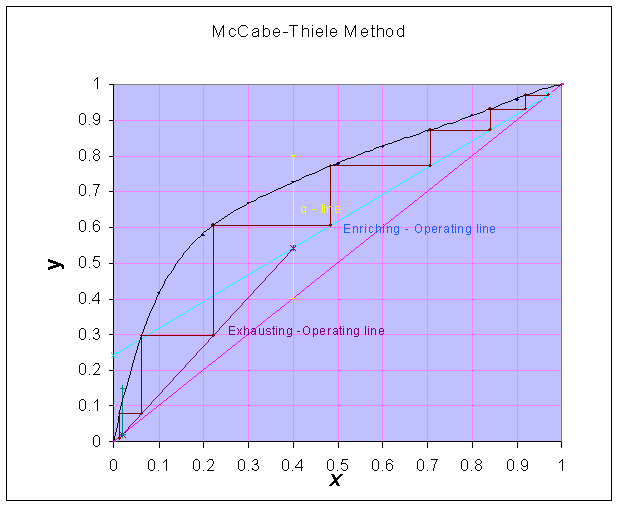### McCabe-Thiele Method

A continuous fractionating column is to be designed for separating 10,000 kg per hour of a liquid mixture containing 40 mole percent methanol and 60 mole percent water into an overhead product containing 97 mole percent methanol and a bottom product having 98 mole percent water. A mole reflux ratio of 3 is used. Calculate (i) moles of overhead product obtained per hour and (ii) number of ideal plates and location of the feed plate if the feed is at its bubble point.

Equilibrium data:

 x 0.1 0.2 0.3 0.4 0.5 0.6 0.7 0.8 0.9 y 0.417 0.579 0.669 0.729 0.78 0.825 0.871 0.915 0.959

Where x = mole fraction of methanol in liquid

And y = mole fraction of methanol in vapor

Data:

Feed rate = 10,000 kg/hr

zF = 0.4

xD = 0.97

xW = 0.02

R = 3

Calculations:

Average molecular weight of feed = 0.4 x 32 + 0.6 x 18 = 23.6

Molal flow rate of feed = 10000/23.6 = 423.73 kmol/hr

Material balance equations:

F = D + W

F zF = D xD + W xW

Where F = feed; D = distillate; W = residue

Substituting for the known quantities,

423.73 = D + W

423.73 x 0.4 = 0.97 D + 0.02 W

0.97 D - 0.02 D = 169.492 - 8.4746

D = 169.492 kmol/hr

Overhead product obtained = 169.492 kmol/hr

(ii) Number of ideal plates:

Slope of 'q - line' = ∞

R = L/D = 3Number of theoretical plates are estimated from the McCabe-Thiele graphical construction method and found to be equal to 6.8

And the location of feed plate is 5th plate (counting from the top)